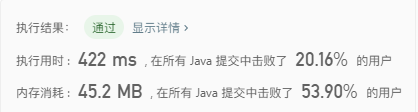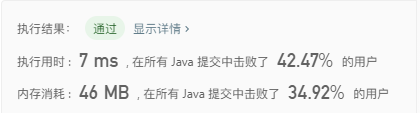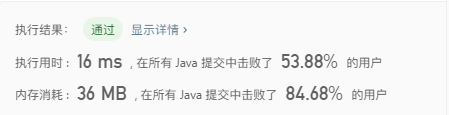## 盛最多水的容器

``````public int maxArea(int[] height) {
if (height.length == 0) {
return 0;
}
int maxArea = Integer.MIN_VALUE;
int tail;
while (head < height.length - 1) {
tail = height.length - 1;
maxArea = Math.max(maxArea, (tail - head) * minSide);
tail --;
}
}
return maxArea;
}````````````public int maxArea(int[] height) {
if (height.length == 0) {
return 0;
}
int maxArea = Integer.MIN_VALUE;
int tail = height.length - 1;
while (head < height.length - 1) {
maxArea = Math.max(maxArea, (tail - head) * minSide);
tail --;
} else {
}
}
return maxArea;
}``````## 最接近的三数之和

``````public int threeSumClosest(int[] nums, int target) {
if (nums.length < 3) {
return target;
}
Arrays.sort(nums);
int closetNum = nums + nums + nums;
for (int i = 0; i < nums.length - 2; i++) {
int l = i + 1;
int r = nums.length - 1;
while (l < r) {
int thisSum = nums[l] + nums[r] + nums[i];
if (Math.abs(thisSum - target) < Math.abs(closetNum - target)) {
closetNum = thisSum;
}
if (thisSum > target) {
r--;
} else if (thisSum < target) {
l++;
} else {
return thisSum;
}
}
}
return closetNum;
}``````